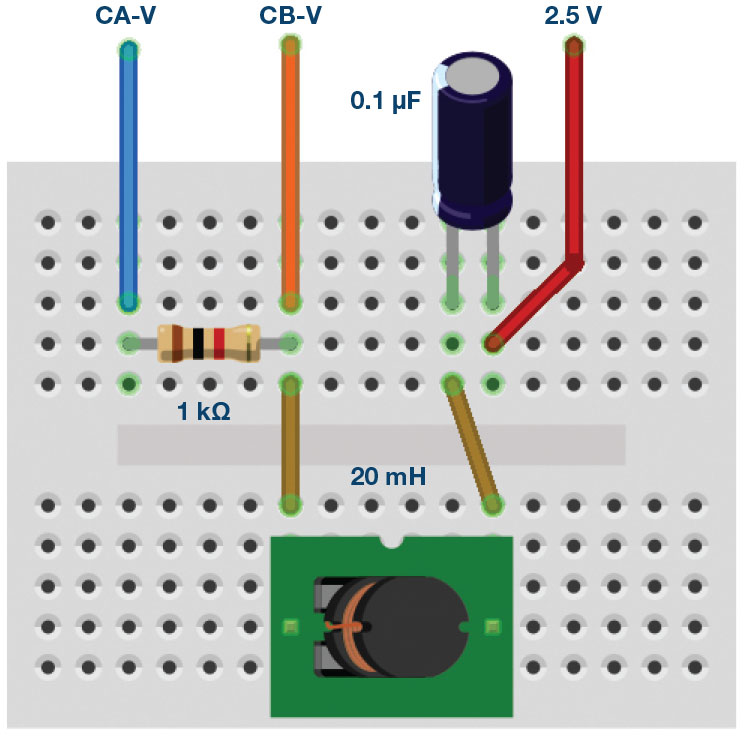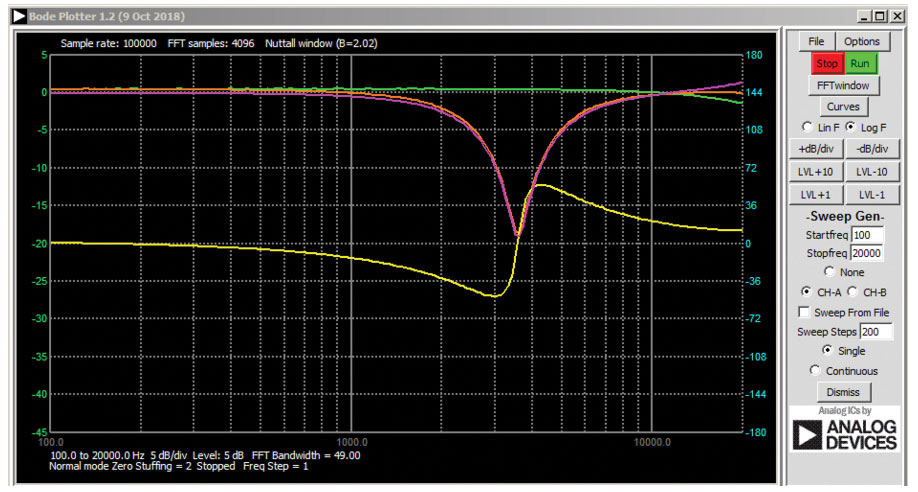# ADALM1000 SMU Training Topic 13: Band Stop Filter

After the introduction of the SMU ADALM1000 in our Analog Dialogue December 2017 article, we want to continue with some small, basic measurements. You can find the previous ADALM1000 article here.Figure 1. A schematic of the ADALM1000.

### Objective:

The objective of this lab activity is to:

1. Construct a band-stop filter by combining a low-pass filter and a high-pass filter. A series LC circuit will be used.
2. Obtain the frequency response of the filter by using the Bode plotter software tool.

### Background:

A band-stop filter, also called a notch or band reject filter, prevents a specific range of frequencies from passing to the output, while allowing lower and higher frequencies to pass with little attenuation. It removes or notches out frequencies between the two cutoff frequencies while passing frequencies outside the cutoff frequencies.

One typical application of a band-stop filter is in audio signal processing, for removing a specific range of undesirable frequencies of sound like noise or hum, while not attenuating the rest. Another application is in the rejection of a specific signal from a range of signals in communication systems.

A band reject filter may be constructed by combining a high-pass RL filter with a roll-off frequency fL and a low-pass RC filter with a roll-off frequency fH, such that:

The higher cutoff frequency is given as:

The lower cutoff frequency is given as:

The bandwidth of frequencies rejected is given by:

All the frequencies below fL and above fH are allowed to pass and the frequencies between are attenuated by the filter. The series combination of an L and C as shown in Figure 2 is such a filter.Figure 2. Band reject filter circuit.

From the previous article on parallel LC resonance, we can also use the formula for the LC resonance to calculate the center frequency of the band-pass filter, the resonant frequency ωo is given by:

or

### Frequency Response:

To show how a circuit responds to a range of frequencies, a plot of the magnitude (amplitude) of the output voltage of the filter as a function of the frequency can be drawn. It is generally used to characterize the range of frequencies in which the filter is designed to operate. Figure 2 shows a typical frequency response of a band-pass filter.Figure 3. Band reject filter frequency response.

### Materials:

• ADALM1000 hardware module
• Resistor R1 1.0 kΩ
• Capacitor C1 0.1 μF (marked 104)
• Inductor L1 (one 20 mH or two 10 mH in series)

### Procedure:

1. Setup the filter circuit as shown in Figure 2 on your solderless breadboard, with the component values R1 = 1 kΩ, C1 = 0.1 μF, L1 = 20 mH.Figure 4. Band reject filter breadboard connections.
2. Set the Channel A AWG Min value to 0.5 and Max value to 4.5 V to apply a 4 V p-p sine wave centered on 2.5 V as the input voltage to the circuit. From the AWG A Mode drop-down menu, select the SVMI mode. From the AWG A Shape drop-down menu, select Sine. From the AWG B Mode drop-down menu, select Hi-Z mode.
3. From the ALICE Curves drop-down menu, select CA-V and CB-V for display. From the Trigger drop-down menu, select CA-V and Auto Level. Set the Hold Off to 2 (ms). Adjust the time base until you have at approximately two cycles of the sine wave on the display grid. From the Meas CA drop-down menu, select P-P under CA-V and do the same for CB. Also, from the Meas CA menu, select A-B Phase.
4. Start with a low frequency (100 Hz) and measure the output voltage CB-V peak to peak from the scope screen. It should be about the same as the Channel A output. Increase the frequency of Channel A in small increments until the peak-to-peak voltage of Channel B is roughly 0.7 times the peak-to-peak voltage for Channel A. Compute 70% of V p-p and obtain the frequency at which this happens on the oscilloscope. This gives the cutoff (roll-off) frequency for the constructed RL time constant of the filter.
5. Continue increasing the frequency of Channel A until the peak-to-peak voltage of Channel B drops to its minimum value. Measure the frequency at which this happens on the oscilloscope. This gives the center frequency for the constructed series LC resonator section of band reject filter, at the lower cutoff and upper cutoff frequencies.

### Frequency Response Plots with the ALICE Bode Plotter:

The ALICE desktop software can display Bode plots, which are graphs of the magnitude and the phase vs. the frequency of a given network. The procedure is as follows:

• Use the band-pass circuit in Figure 2, with R1 = 1.0 kΩ, C1 = 0.1 μF, and L1 = 20 mH so that we can sweep the input frequency from 500 Hz to 12,000 Hz and plot the signal amplitude of both Channel A and Channel B and the relative phase angle between Channel B and Channel A.
• With the circuit connected to the ALM1000, as in Figure 2, start the ALICE Desktop software.
• Open the Bode Plotting tool. Under the Curves menu, select CA-dBV, CB-dBV, and Phase B-A.
• Under the Options menu, change the setting for zero-stuffing to 2.
• Set the AWG Channel A Min value to 1.086 and the Max value to 3.914. This will be a 1 V rms (0 dBV) amplitude centered on the 2.5 V middle of the analog input range. Set AWG A mode to SVMI and Shape to Sine. Set AWG Channel B to Hi-Z mode. Be sure the Sync AWG check box is selected.
• Use the Start Frequency entry to set the frequency sweep to start at 100 Hz and use the Stop Frequency entry to the sweep to stop at 20,000 Hz. Under Sweep Gen, select CHA as the channel to sweep. Also use the Sweep Steps entry to enter the number of frequency steps, using 200 as the number.

You should now be able to press the green Run button and run the frequency sweep. After the sweep is completed (this could take a few seconds for 200 points), you should see something like the screenshot in Figure 5. You may want to use the LVL and dB/div buttons to optimize the plots to best fit the screen grid.

Record the results and save the Bode plot as a screenshot.Figure 5. Bode analyzer settings band-stop filter.

### Questions:

1. Compute the cutoff frequencies for each band reject filter constructed using the formula in Equation 1 and 2. Compare these theoretical values to the ones obtained from the experiment and provide suitable explanation for any differences.

You can find the answers at the StudentZone blog.

### Notes:

As in all the ALM labs, we use the following terminology when referring to the connections to the ALM1000 connector and configuring the hardware. The green shaded rectangles indicate connections to the ADALM1000 analog I/O connector. The analog I/O channel pins are referred to as CA and CB. When configured to force voltage/measure current, -V is added (as in CA-V) or when configured to force current/measure voltage, -I is added (as in CA-I). When a channel is configured in the high impedance mode to only measure voltage, -H is added (as in CA-H).

Scope traces are similarly referred to by channel and voltage/current, such as CA-V and CB-V for the voltage waveforms, and CA-I and CB-I for the current waveforms.

We are using the ALICE Rev 1.1 software for those examples here.

File: alice-desktop-1.1-setup.zip. Please download here.

The ALICE Desktop software provides the following functions:

• A 2-channel oscilloscope for time domain display and analysis of voltage and current waveforms.
• The 2-channel arbitrary waveform generator (AWG) controls.
• The X and Y display for plotting captured voltage and current vs. voltage and current data, as well as voltage waveform histograms.
• The 2-channel spectrum analyzer for frequency domain display and analysis of voltage waveforms.
• The Bode plotter and network analyzer with built-in sweep generator.
• An impedance analyzer for analyzing complex RLC networks and as an RLC meter and vector voltmeter.
• A dc ohmmeter measures unknown resistance with respect to known external resistor or known internal 50 Ω.
• Board self-calibration using the AD584 precision 2.5 V reference from the ADALP2000 analog parts kit.
• ALICE M1K voltmeter.
• ALICE M1K meter source.
• ALICE M1K desktop tool.

For more information, please look here.

Note: You need to have the ADALM1000 connected to your PC to use the software.Figure 6. ALICE desktop 1.1 menu.